Courses

# Chemistry - 2016 Past Year Paper

## 60 Questions MCQ Test IIT JAM Chemistry Past Year Papers | Chemistry - 2016 Past Year Paper

Description
This mock test of Chemistry - 2016 Past Year Paper for IIT JAM helps you for every IIT JAM entrance exam. This contains 60 Multiple Choice Questions for IIT JAM Chemistry - 2016 Past Year Paper (mcq) to study with solutions a complete question bank. The solved questions answers in this Chemistry - 2016 Past Year Paper quiz give you a good mix of easy questions and tough questions. IIT JAM students definitely take this Chemistry - 2016 Past Year Paper exercise for a better result in the exam. You can find other Chemistry - 2016 Past Year Paper extra questions, long questions & short questions for IIT JAM on EduRev as well by searching above.
QUESTION: 1

Solution:
QUESTION: 2

Solution:
QUESTION: 3

### The mechanism of the following transformation involves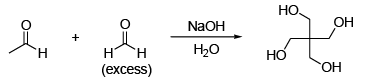Solution:
QUESTION: 4

The most basic amino acid among the following is

Solution:
QUESTION: 5

The crystal field stabilization energy (CFSE) in [Mn(H2O)6]2+ is

Solution:
QUESTION: 6

Indicator used in redox titration is

Solution:
QUESTION: 7

Among the following, the compound that has the lowest degree of ionic character is

Solution:
QUESTION: 8

The correct order of entropy for various states of CO2 is

Solution:
QUESTION: 9

The coordination numbers of Cs+ and Cl ions in the CsCl structure, respectively, are

Solution:
QUESTION: 10

Determinant of a square matrix is always

Solution:
QUESTION: 11

The correct order of 1H NMR chemical shift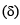values for the labeled methyl groups in the following compound is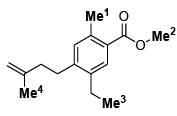Solution:
QUESTION: 12

Among the following, the most stable conformation of meso-2,3-dibromobutane is

Solution:
QUESTION: 13

The major products X and Y in the following reaction sequence are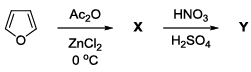Solution:
QUESTION: 14

The major product formed in the reaction of butanenitrile with phenylmagnesium bromide followed by acidification is

Solution:
QUESTION: 15

An organic compound on reaction with 2,4-dinitrophenylhydrazine (2,4-DNP) gives a yellow precipitate. It also gives silver mirror on reaction with ammoniacal AgNO3. It gives an alcohol and sodium salt of a carboxylic acid on reaction with concentrated NaOH. It yields benzene-1,2-dicarboxylic acid on heating with alkaline KMnO4. The structure of the compound among the following is

Solution:
QUESTION: 16

The major products X and Y in the following reaction sequence are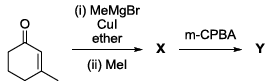Solution:
QUESTION: 17

The TRUE statement about [Cu(H2O)6]2+ is

Solution:
QUESTION: 18

The complexes [Pt(CN)4]2– and [NiCl4]2–, respectively, are

Solution:
QUESTION: 19

The value of ‘x’ in [Cu(CO)x]+ such that it obeys the 18 electron rule is

Solution:
QUESTION: 20

The correct order of νNO (cm–1) in the following compounds is

Solution:
QUESTION: 21

The red color of ruby is due to

Solution:
QUESTION: 22

The final products in the reaction of BF3 with water are

Solution:
QUESTION: 23

The correct order of bond angles in BF3, NH3, NF3 and PH3 is

Solution:
QUESTION: 24

The maximum of a function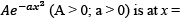Solution:
QUESTION: 25

At 298 K, 0.1 mol of ammonium acetate and 0.14 mol of acetic acid are dissolved in 1 L of water. The pH of the resulting solution is
[Given: pKa of acetic acid is 4.75]

Solution:
QUESTION: 26

An electrochemical cell consists of two half-cell reactions
AgCl(s) + e → Ag(s) + Cl(aq)
Cu(s) → Cu2+(aq) + 2e

The mass of copper (in grams) dissolved on passing 0.5 A current for 1 hour is
[Given: atomic mass of Cu is 63.6; F = 96500 C mol–1]

Solution:
QUESTION: 27

For a zero order reaction, the half-life depends on the initial concentration [C0] of the reactant as

Solution:
QUESTION: 28

The effective nuclear charge of helium atom is 1.7. The first ionization energy of helium atom in eV is

Solution:
QUESTION: 29

The relationship between the van der Waals ‘b’ coefficient of N2 and O2 is

Solution:
QUESTION: 30

From the kinetic theory of gases, the ratio of most probable speed (Cmp) to root mean square speed (Crms) is

Solution:
*Multiple options can be correct
QUESTION: 31

The correct statement(s) about the following species is(are)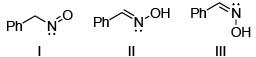Solution:
*Multiple options can be correct
QUESTION: 32

Consider the following reaction: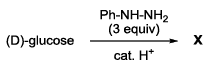Among the following, the compound(s) whose osazone derivative(s) will have the same melting point as that of X is(are)

Solution:
*Multiple options can be correct
QUESTION: 33

The appropriate reagents required for carrying out the following transformation are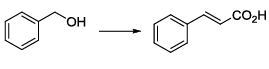Solution:
*Multiple options can be correct
QUESTION: 34

The appropriate reagents required for carrying out the following transformation are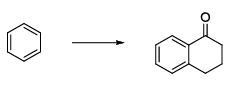Solution:
*Multiple options can be correct
QUESTION: 35

The protein(s) that belong to the class of blue copper proteins is(are)

Solution:
*Multiple options can be correct
QUESTION: 36

The ion(s) that exhibit only charge transfer bands in the absorption spectra (UV-visible region) is(are)

Solution:
*Multiple options can be correct
QUESTION: 37

The type(s) of interaction(s) that hold layers of graphite together is(are)

Solution:
*Multiple options can be correct
QUESTION: 38

TRUE statement(s) about Langmuir isotherm is(are)

Solution:
*Multiple options can be correct
QUESTION: 39

The 3pz orbital has

Solution:
*Multiple options can be correct
QUESTION: 40

The diatomic molecule(s) that has(have) two π-type bonds is(are)

Solution:
*Answer can only contain numeric values
QUESTION: 41

Among the following, the number of molecules that are aromatic is __.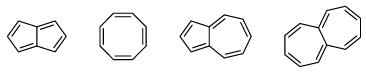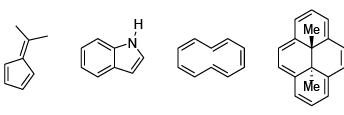Solution:
*Answer can only contain numeric values
QUESTION: 42

The number of all possible isomers for the molecular formula C6H14 is __.

Solution:
*Answer can only contain numeric values
QUESTION: 43

Hydrolysis of 15.45 g of benzonitrile produced 10.98 g of benzoic acid. The percentage yield of acid formed is __.

Solution:
*Answer can only contain numeric values
QUESTION: 44

Acetic acid content in commercial vinegar was analyzed by titrating against 1.5 M NaOH solution. A 20 mL vinegar sample required 18 mL of titrant to give endpoint. The concentration of acetic acid in the vinegar (in mol L–1) is __.

Solution:
*Answer can only contain numeric values
QUESTION: 45

The bond order of Be2 molecule is __.

Solution:
*Answer can only contain numeric values
QUESTION: 46

The number of P–H bonds in hypophosphorus acid is __.

Solution:
*Answer can only contain numeric values
QUESTION: 47

The isotope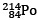undergoes one alpha and one beta particle emission sequentially to form an isotope “X”. The number of neutrons in “X” is __.

Solution:
*Answer can only contain numeric values
QUESTION: 48

In a diffraction experiment with X-rays of wavelength 1.54 Å, a diffraction line corresponding to 2θ = 20.8° is observed. The inter-planar separation in Å is __.

Solution:
*Answer can only contain numeric values
QUESTION: 49

The potential energy of interaction between two ions in an ionic compound is given by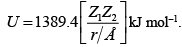Assuming that CaCl2 is linear molecule of length 5.6 Å, the potential energy for CaCl2 molecule in kJ mol-1 is __.

Solution:
*Answer can only contain numeric values
QUESTION: 50

The enthalpy of formation for CH4(g), C(g) and H(g) are –75, 717 and 218 kJ mol–1, respectively. The enthalpy of the C–H bond in kJ mol–1 is __.

Solution:
*Answer can only contain numeric values
QUESTION: 51

Specific rotation of the (R)-enantiomer of a chiral compound is 48. The specific rotation of a sample of this compound which contains 25% of (S)-enantiomer is ___.

Solution:
*Answer can only contain numeric values
QUESTION: 52

Among the following, the number of compounds, which can participate as ‘diene’ component in a Diels-Alder reaction is __.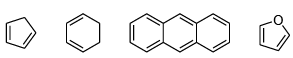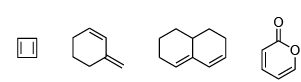Solution:
*Answer can only contain numeric values
QUESTION: 53

Among the following, the number of molecules that possess C2 axis of symmetry is __.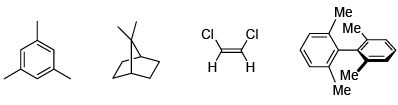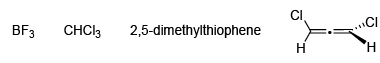Solution:
*Answer can only contain numeric values
QUESTION: 54

Effective nuclear charge for 3d electron in vanadium (atomic number = 23) according to Slater’s rule is __.

Solution:
*Answer can only contain numeric values
QUESTION: 55

The total number of isomers possible for the molecule [Co(NH3)4Cl(NO2)]+ is __.

Solution:
*Answer can only contain numeric values
QUESTION: 56

The bond angle in PBr3 is 101°. The percent ‘s’ character of the central atom is __.

Solution:

From Bent’s Rule equation[ cosx = s/s-1( where x is an bond angle and s is the % s character in the bond)], it is clear that if we increase bond angle, the %S Character will be maximum.

*Answer can only contain numeric values
QUESTION: 57

Cu(s) + 4 H+(aq) + 2NO3(aq) → 2NO2(g) + Cu2+(aq) + 2H2O(l)
In the above reaction at 1 atm and 298 K, if 6.36 g of copper is used. Assuming ideal gas behavior, the volume of NO2 produced in liters is __.
[Given: atomic mass of Cu is 63.6; R = 0.0821 L atm K–1 mol–1]

Solution:
*Answer can only contain numeric values
QUESTION: 58

The ΔH° for the reaction CO(g) + ½O2(g) → CO2(g) at 400 K in kJ mol–1 is __.
Given at 298 K: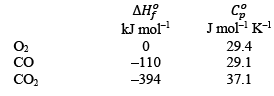Solution:
*Answer can only contain numeric values
QUESTION: 59

The rate constants for a reaction at 300 and 350 K are 8 and 160 L mol–1 s–1, respectively. The activation energy of the reaction in kJ mol–1 is __.
[Given R = 8.314 J K–1 mol–1]

Solution:
*Answer can only contain numeric values
QUESTION: 60

A 10 L flask containing 10.8 g of N2O5 is heated to 373 K, which leads to its decomposition according to the equation 2 N2O5(g) → 4 NO2(g) + O2(g). If the final pressure in the flask is 0.5 atm, then the partial pressure of O2(g) in atm is __.
[Given R = 0.0821 L atm K–1 mol–1]

Solution: